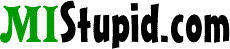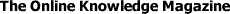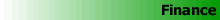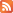# Compound Interest

Interest which is calculated not only on the initial principal but also the accumulated interest of prior periods. Compound interest differs from simple interest in that simple interest is calculated solely as a percentage of the principal sum.

Compounding Interest Calculator
 Initial Deposit Amount: \$ Monthly Contribution: \$ Assumed Annual Return: % Number of years:

 Home | Advertising | Donate | Contribute | Link to Us | Privacy | Contact | About |© 2001-2023 MIStupid.com